# Class 9 RD Sharma Solutions – Chapter 12 Heron’s Formula- Exercise 12.2

• Last Updated : 30 Apr, 2021

### Question 1. Find the area of the quadrilateral ABCD in which AB = 3 cm, BC = 4 cm, CD = 4 cm, DA = 5 cm and AC = 5 cm.

Solution: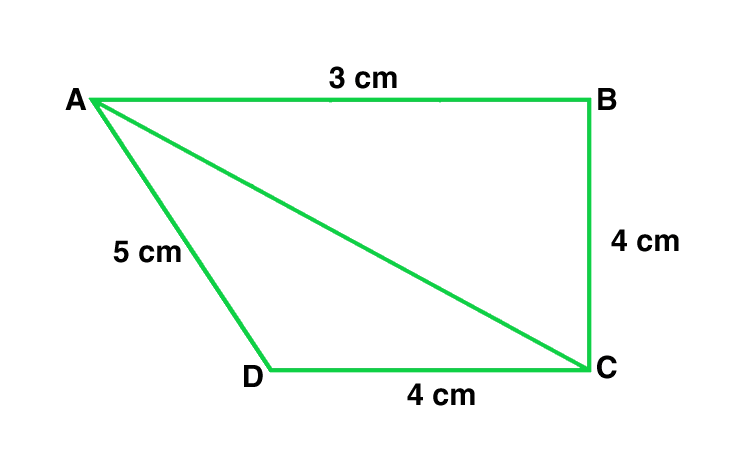In triangle ABC of Quadrilateral ABCD

AC2 = BC2 + AB2

25 = 9 + 16

Hence, triangle ABC is a right angle triangle right-angled at point R

As we know that, Area of triangle ABC = 12 × AB × BC

= 1/2 × 3 × 4 = 6 cm2

Perimeter = 2s = AC + CD + DA

2s = 5 cm+ 4 cm+ 5 cm

2s = 14 cm

s = 7 cm

By using Heron’s Formula,

Area of triangle = √s x (s – a) x (s – b) x (s – c)

= √7 x (7 – 5) x (7 – 4) x (7 – 5) = 9.16 cm2

Area of ABCD = Area of ABC + Area of CAD

= (6 + 9.16) cm2 = 15.16 cm2

### Question 2. The sides of a quadrilateral field, taken in order are 26 m, 27 m, 7 m, 24 m respectively. The angle contained by the last two sides is a right angle. Find its area.

Solution: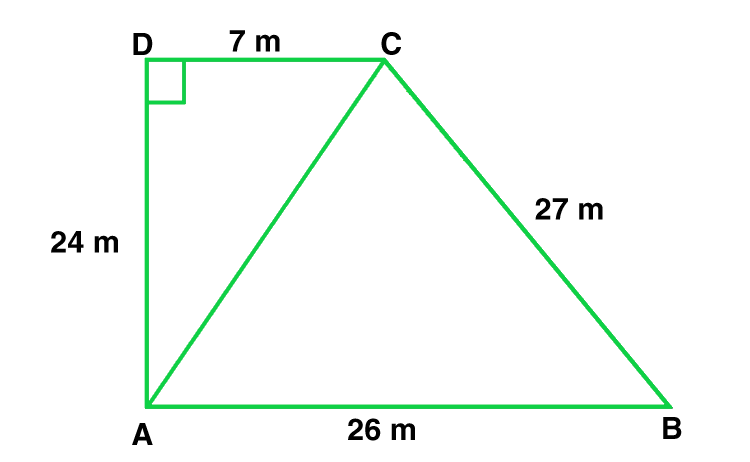Given that,

AB = 26 m, BC = 27 m, CD = 7 m, DA = 24 m

Diagonal AC is joined.

By applying Pythagoras theorem,

AC2 = 142  + 72

AC = 25 m

Now Area of triangle ABC,

Perimeter = 2s = AB + BC + CA

2s = 26 m + 27 m + 25 m

s = 39 m

By using Heron’s Formula,

Area of triangle ABC = √s x (s – a) x (s – b) x (s – c)

= √ 39 x (39 – 26) x (39 – 27) x (39 – 25) = 291.84 m2

Thus, Area of a triangle is 291.84 m2

Now for Area of triangle ADC,

Perimeter = 2S = AD + CD + AC

= 25 m + 24 m + 7 m

S = 28 m

By using Heron’s Formula,

Area of triangle ADC = √s x (s – a) x (s – b) x (s – c)

= √ 28 x (28 – 24) x (28 – 7) x (28 – 25) = 84 m2

Thus, Area of a triangle is 84m2

Therefore, Area of rectangular field ABCD

= Area of triangle ABC + Area of triangle ADC

= 291.84 + 84 = 375.8 m2

### Question 3. The sides of a quadrilateral, taken in order as 5 m, 12 m, 14 m, 15 m respectively, and the angle contained by first two sides is a right angle. Find its area.

Solution: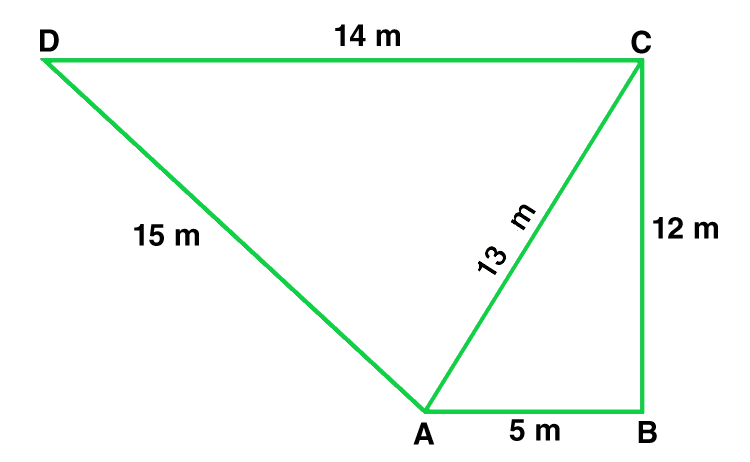Given that,

AB = 5 m, BC = 12 m, CD =14 m and DA = 15 m

Join AC

Now area of triangle ABC = ½ × AB × BC

= 1/2× 5 × 12 = 30 m2

In triangle ABC,

By applying Pythagoras theorem

AC2 = AB2 + BC2

AC = √52 + 122 = 13 m

Perimeter = 2s = AD + DC + AC

2s = 15 m + 14 m + 13 m

s = 21 m

By using Heron’s Formula,

Area of triangle = √s x (s – a) x (s – b) x (s – c)

= √ 21 x (21 – 15) x (21 – 14) x (21 – 13) = 84 m2

Area of quadrilateral ABCD = Area of triangle ABC + Area of triangle ADC

= (30 + 84) m2 = 114 m2

### Question 4. A park in the shape of a quadrilateral ABCD, has angle C = 90°, AB = 9 m, BC = 12 m, CD = 5 m, AD = 8 m. How much area does it occupy?

Solution: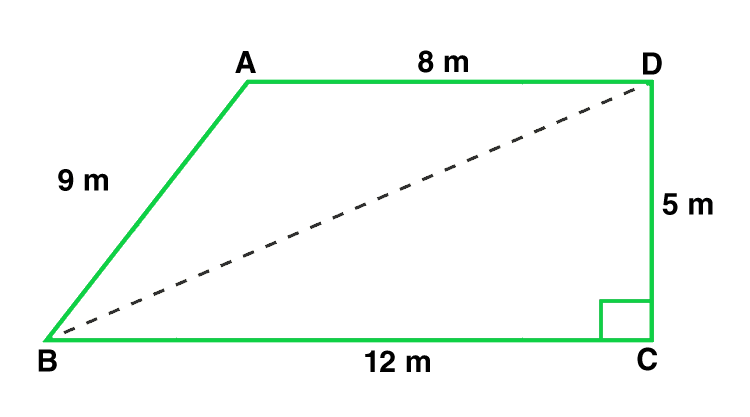Given that,

Sides of a quadrilateral are AB = 9 m, BC = 12 m, CD = 5 m, DA = 8 m.

In triangle BCD, apply Pythagoras theorem

BD2 = BC2 + CD2

BD2 = 122 + 52

BD = 13 m.

Area of triangle BCD = 1/2 × BC × CD

= 1/2 × 12 × 5 = 30 m2

Now, in triangle ABD

Perimeter = 2s = 9 m + 8m + 13m

s = 15 m

By using Heron’s Formula,

Area of triangle = √s x (s – a) x (s – b) x (s – c)

= √ 15 x (15 – 9) x (15 – 8) x (15 – 13) = 35.49 m2

Area of quadrilateral ABCD = Area of triangle ABD + Area of triangle BCD

= (35.496 + 30) m2 = 65.5m2.

### Question 5. Two parallel sides of a trapezium are 60 m and 77 m and the other sides are 25 m and 26 m. Find the area of the trapezium?

Solution: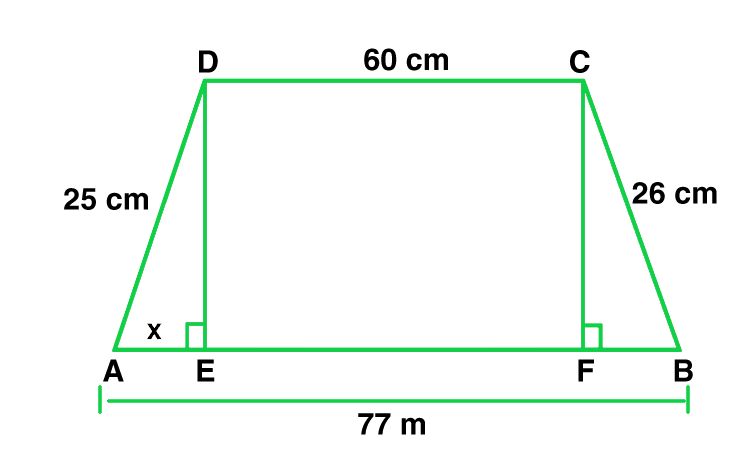Given that,

Two parallel sides of trapezium are AB = 77 m and CD = 60 m

The other two parallel sides of trapezium are BC = 26 m, AD = 25m

DE is perpendicular to AB and also, CF is perpendicular to AB

Therefore, DC = EF = 60 m

Let AE = x

therefore, BF = 77 – 60 – x

BF = 17 – x

By using Pythagoras theorem,

DE2 = 252 − x2

In triangle BCF,

By using Pythagoras theorem,

CF2 = BC2 − BF2

CF2 = 262 − (17 − x)2

Here, DE = CF

therefore, DE2 = CF2

252 − x2 = 262 − (17 − x)2

252 − x2 = 262 − (172 − 34x + x2)

252 − x2 = 262 − 172 + 34x + x2

252 = 262 − 172 + 34x

x = 7

DE2 = 252 − x2

DE = √625 – 49

DE = 24 m

Area of trapezium = 1/2 × (60 + 77) × 24

Hence, Area of trapezium = 1644 m2

### Question 6. Find the area of a rhombus whose perimeter is 80 m and one of whose diagonal is 24 m.

Solution: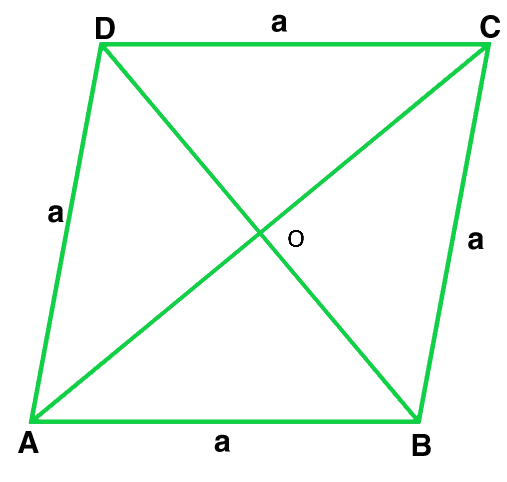Given that,

Perimeter of a rhombus = 80 m and AC = 24 m

As we know that, Perimeter of a rhombus = 4 × side = 4 × a

4 × a = 80 m

a = 20 m

OA = 1/2 × AC

OA = 12 m

In triangle AOB,

OB2 = AB2 − OA2

OB2 = 202 − 122

OB = 16 m

Also, OB = OD because diagonal of rhombus bisect each other at 90°

Therefore, BD = 2 OB = 2 × 16 = 32 m

Area of rhombus = 1/2 × BD × AC

Area of rhombus = 1/2 × 32 × 24

Area of rhombus = 384 m2

### Question 7. A rhombus sheet, whose perimeter is 32 m and whose diagonal is 10 m long, is painted on both the sides at the rate of Rs 5 per meter square. Find the cost of painting.

Solution: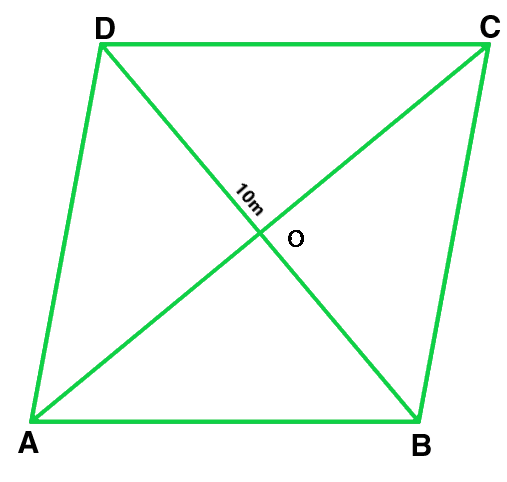Given that,

Perimeter of a rhombus = 32 m

As we know that, Perimeter of a rhombus = 4 × side

4 × side = 32 m

4 × a = 32 m

a = 8 m

AC = 10 m                     (Given)

OA = 12 × AC

OA = 12 × 10 = 5 m

By using Pythagoras theorem,

OB2 = AB2 − OA2

OB2 = 82 − 52

OB = √39 m

BD = 2 × OB

BD = 2√39 m

Area of the sheet = 1/2 × BD × AC

Area of the sheet = 1/2 ×2√39 × 10

Therefore, cost of printing on both sides at the rate of Rs. 5 per m2

= Rs 2 × 10√39 × 5 = Rs. 625

### Question 8. Find the area of the quadrilateral ABCD in which AD = 24 cm, angle BAD = 90° and BCD forms an equilateral triangle whose each side is equal to 26 cm. [Take √3 = 1.73]

Solution: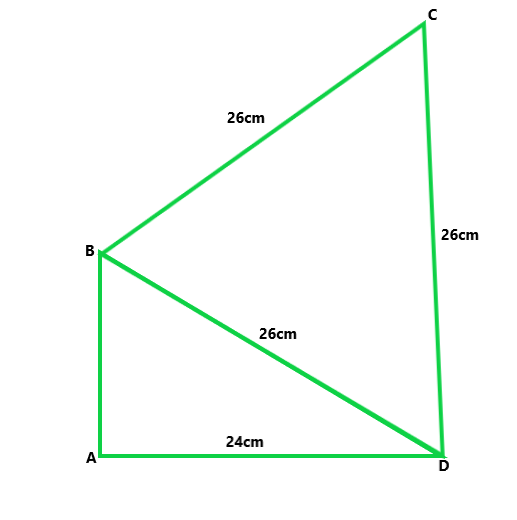Given that, in a quadrilateral ABCD in which AD = 24 cm,

BCD is an equilateral triangle and the sides BC = CD = BD = 26 cm.

In triangle BAD, by applying Pythagoras theorem,

BA2 = 262 + 242

BA = √100 = 10 cm

= 1/2 × 10 × 24 = 120 cm2

Area of the equilateral triangle = √3/4 × side

Area of the equilateral triangle QRS = √3/4 × 26

Area of the equilateral triangle BCD = 292.37 cm2

Therefore, the area of quadrilateral ABCD = Area of triangle BAD + Area of the triangle BCD

The area of quadrilateral ABCD = 120 + 292.37 = 412.37 cm2

### Question 9. Find the area of quadrilateral ABCD in which AB = 42 cm, BC = 21 cm, CD = 29 cm, DA = 34 cm and the diagonal BD = 20 cm.

Solution: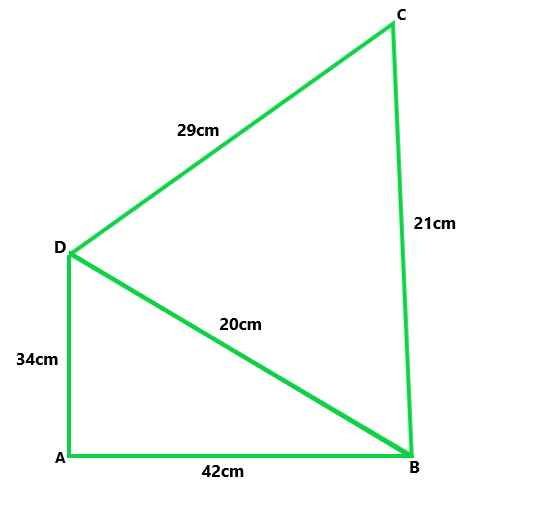Given that,

AB = 42 cm, BC = 21 cm, CD = 29 cm, DA = 34 cm, and the diagonal BD = 20 cm.

Now, for the area of triangle ABD,

Perimeter of triangle ABD 2s = AB + BD + DA

2s = 34 cm + 42 cm + 20 cm

s = 48 cm

By using Heron’s Formula,

Area of triangle ABD = √s x (s – a) x (s – b) x (s – c)

= √ 48 x (48 – 42) x (48 – 20) x (48 – 34) = 33 6cm2

Now, for the area of triangle BCD,

Perimeter of triangle BCD 2s = BC + CD + BD

2s = 29cm + 21cm + 20cm

s = 35 cm

By using Heron’s Formula,

Area of triangle BCD = √s x (s – a) x (s – b) x (s – c)

= √ 35 x (14) x (6) x (15) = 210 cm2

Therefore, Area of quadrilateral ABCD = Area of triangle ABD + Area of triangle BCD

Area of quadrilateral ABCD = 336 + 210

Area of quadrilateral ABCD = 546 cm2

### Question 10. Find the perimeter and the area of the quadrilateral ABCD in which AB = 17 cm, AD = 9 cm, CD = 12 cm, AC = 15 cm and angle ACB = 90°.

Solution: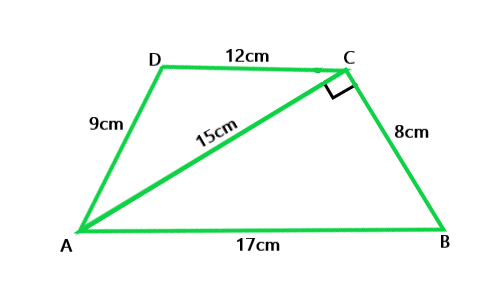Given that,

The sides of the quadrilateral ABCD in which AB = 17 cm, AD = 9 cm, CD = 12 cm, AC = 15 cm and an angle ACB = 90°

By using Pythagoras theorem,

BC2 = AB2 − AC2

BC2 = 172 − 152

BC = 8 cm

Now, area of triangle ABC = ½ × AC × BC

= 1/2 × 8 × 15 = 60 cm2

Now, for the area of triangle ACD

Perimeter of triangle ACD 2s = AC + CD + AD

2s = 15 + 12 + 9

s = 18 cm

By using Heron’s Formula,

Area of triangle ACD = √s x (s – a) x (s – b) x (s – c)

= √ 18 x (3) x (6) x (9) = 54 cm2

Area of quadrilateral ABCD = Area of triangle ABC + Area of triangle ACD

= 60 cm2 + 54 cm2

Hence, Area of quadrilateral ABCD = 114 cm2

### Question 11. The adjacent sides of a parallelogram ABCD measure 34 cm and 20 cm, and the diagonal AC measures 42 cm. Find the area of parallelogram.

Solution: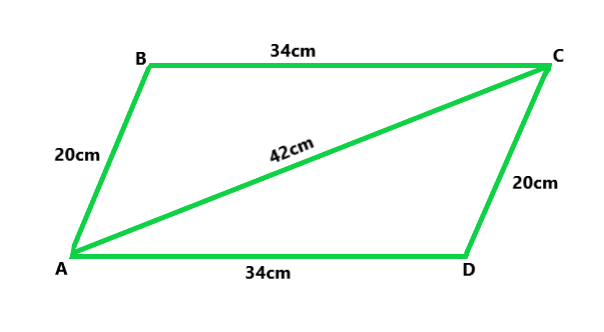Given that,

The adjacent sides of a parallelogram ABCD measures 34 cm and 20 cm, and the diagonal AC measures 42 cm.

Area of the parallelogram = Area of triangle ADC + Area of triangle ABC

As we know that, Diagonal of a parallelogram divides into two congruent triangles

Therefore, Area of the parallelogram = 2 × (Area of triangle ABC)

Now, for area of triangle ABC

Perimeter = 2s = AB + BC + CA

2s = 34 cm + 20 cm + 42 cm

s = 48 cm

By using Heron’s Formula,

Area of triangle ABC = √s x (s – a) x (s – b) x (s – c)

= √ 48 x (14) x (28) x (6) = 336 cm2

Therefore, area of parallelogram ABCD = 2 × (Area of triangle ABC)

Area of parallelogram = 2 × 336 cm2

Hence, Area of parallelogram ABCD = 672 cm2

### Question 12. Find the area of the blades of the magnetic compass shown in the figure given below:

Solution: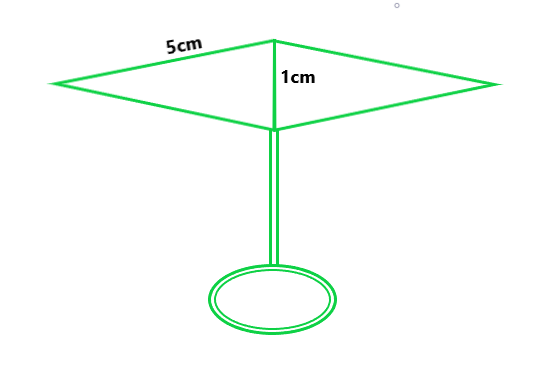From the figure we conclude that, Area of the blades of magnetic compass = Area of triangle ADB + Area of triangle CDB

Now, for the area of triangle ADB,

Perimeter = 2s = AD + DB + BA

2s = 5 cm + 1 cm + 5 cm

s = 5.5 cm

By using Heron’s Formula,

Area of triangle DEF = √s x (s – a) x (s – b) x (s – c)

= √ 5.5 x (0.5) x (4.5) x (0.5) = 2.49 cm2

Also, area of triangle ADB = Area of triangle CDB

Therefore, area of the blades of the magnetic compass = 2 × area of triangle ADB

Area of the blades of the magnetic compass = 2 × 2.49

Hence, Area of the blades of the magnetic compass = 4.98 cm2

### Question 13. A hand fan is made by sticking 10 equal size triangular strips of two different types of paper as shown in the figure. The dimensions of equal strips are 25 cm, 25 cm and 14 cm. Find the area of each type of paper needed to make the hand fan.

Solution: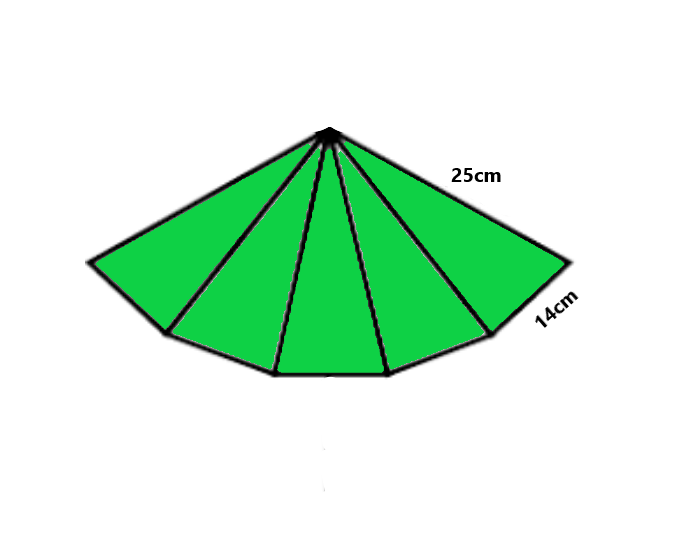Given that,

The sides of AOB,

AO = 25 cm,

OB = 25 cm,

BA = 14 cm.

Area of each strip = Area of triangle AOB

Now, for the area of triangle AOB

Perimeter = AO + OB + BA

2s = 25 cm +25 cm + 14 cm

s = 32 cm

By using Heron’s Formula,

Area of triangle AOB = √s x (s – a) x (s – b) x (s – c)

= √ 32 x (7) x (4) x (18) = 168 cm2

Also, area of each type of paper needed to make a fan = 5 × Area of triangle AOB

Area of each type of paper needed to make a fan = 5 × 168 cm2

Area of each type of paper needed to make a fan = 840 cm2

### Question 14. A triangle and a parallelogram have the same base and the same area. If the sides of the triangle are 13 cm, 14 cm, and 15 cm and the parallelogram stands on the base 14 cm, find the height of a parallelogram.

Solution:

Given that,

DC = 15 cm,

CE = 13 cm,

ED = 14 cm.

Let’s assume that the h be the height of parallelogram ABCD,

Now, for the area of triangle DCE.

Perimeter = DC + CE + ED

2s = 15 cm + 13 cm + 14 cm

s = 21 cm

By using Heron’s Formula,

Area of triangle AOB = √s x (s – a) x (s – b) x (s – c)

= √ 21 x (7) x (8) x (6) = 84 cm2

Also, area of triangle DCE = Area of parallelogram ABCD = 84 cm2

24 × h = 84 cm2

h = 6 cm.

My Personal Notes arrow_drop_up